# Properties of Line Integrals question

• I
• WMDhamnekar

#### WMDhamnekar

MHB
TL;DR Summary
Let ##f(x,y) =\frac{-y}{x^2+y^2} \hat{i} + \frac{x}{x^2+y^2}\hat{j}## for all ## (x,y) \not= (0,0),## and ##C: x= \cos {(t)}, y = \sin{(t)} , 0\leq t \leq 2\pi.##

(a) Show that ##f = \nabla F,## for ##F(x,y)= \tan^{-1}{\bigg(\frac{y}{x}\bigg)}##

(b)Show that ## \displaystyle\oint_C f\cdot dr = 2\pi.## Does this contradict the following corollary 4.6 ? If yes, explain, If no, explain.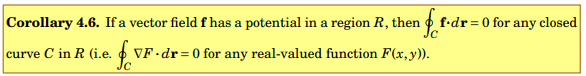I don't have any idea to answer these questions. I am working on it by searching the reference books where similar questions have been solved by authors. Meanwhile, any member of Physics Forums may help me in answering these questions.

Last edited:

Hint for a) ## \vec f(x,y) = f_x (x,y) \hat i + f_y (x,y) \hat j = \dfrac{\partial F(x,y)}{\partial x} \hat i + \dfrac{\partial F(x,y)}{\partial y} \hat j ##

It is just a matter of using the definition of gradient. Now one just have to do some partial derivatives and some algebra.

b) Can you draw in a x-y coordinate system the curve C? Is the given F(x,y) defined for all points on and inside this curve?

Answer to (a) ##f(x,y) = \frac{-y}{x^2+y^2}\hat{i} + \frac{x}{x^2+y^2}\hat{j}## has a potential ##F(x,y) =\arctan{y/x}##
Now, ##\nabla F = \frac{x}{x^2+y^2}\hat{j}= f(x,y) \because \frac{-y}{x^2+y^2}\hat{i} =0 ## for y= sin(t) where 0≤t ≤2π

Answer to (b) ##\displaystyle\oint_C f\cdot dr = \displaystyle\int_0^{2\pi} 1dt= 2\pi##. The answer is nonzero. So it contradicts with the given corollary 4.6.

Last edited:
In my opinion,

Your result for b) does not contradict corollary 4.6, look at what conditions must be fulfilled in order for that corollary to apply. Are those conditions met in this problem? Does an equilateral triangle contradict Pythagoras theorem?

For a) you have not done anything I told you to do, you need to evaulate the partial derivatives of F(x,y) and show that those indeed match the given components of your function f(x,y).

Last edited by a moderator:

##\frac{\partial{\arctan{(\frac{y}{x})}}}{\partial{x}}= \frac{-y}{x^2+y^2}, \frac{\partial{\arctan{(\frac{y}{x})}}}{\partial{y}}= \frac{x}{x^2+y^2}##
So, we can say ##f=\nabla F ## for F(x,y) = tan-1(y/x)

Answer to (b) ##\displaystyle\oint_C f \cdot dr = 2\pi ## f(x,y) is valid for all (x,y ) ≠ (0,0).
Now , In the following cases -sin(t) = 0, when t =0, π, 2π. cos(t) =0 when t=π/2, 3π/2.
Now, how to use that information here?
I am unable to graph this f(x,y) in 2D? If you show me how does it look in 2d, It would be better to grasp your answer.

I wrote that you should graph the curve C, not f(x,y)...
Is F(x,y) defined for all points on the curve C and inside the curve C?
Look carefully at the expression for F(x,y) - what x and y values makes it not being defined?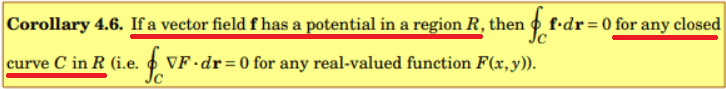I wrote that you should graph the curve C, not f(x,y)...
Is F(x,y) defined for all points on the curve C and inside the curve C?
Look carefully at the expression for F(x,y) - what x and y values makes it not being defined?
View attachment 303591
F(x,y)= tan-1(y/x) is undefined for (x,y)=(0,0). So, How can we say it doesn't contradict corollary 4.6?

F(x,y)= tan-1(y/x) is undefined for (x,y)=(0,0).

There are more points for which it is not defined.

So, How can we say it doesn't contradict corollary 4.6?
What are the requirements for corollary 4.6?

•WMDhamnekar
Equilateral triangle doesn't contradict Pythagoras theorem.
The requirement of corollary 4.6 is ##\displaystyle\oint_C \nabla F \cdot dr =0 ## which is not fulfilled in our case.

So, you mean to say, that's why it doesn't contradict the given corollary. If that logic is correct, Okay. I agrre with you.

f(x,y) does not have a potential for all the points in the region which C encloses, because F(x,y) is not defined when x = 0 and x=0 is indeed happening inside (and on) C. Therefore, the corollary does not apply. It is not contradicted, because its requirements are not fulfilled so there is nothing to be contradicted.

Last edited by a moderator:
•Delta2 and WMDhamnekar
I wrote that you should graph the curve C, not f(x,y)...
Is F(x,y) defined for all points on the curve C and inside the curve C?
Look carefully at the expression for F(x,y) - what x and y values makes it not being defined?
View attachment 303591
I think the shaded region(drawn red lines) in the following graph is the line integral intended in this question.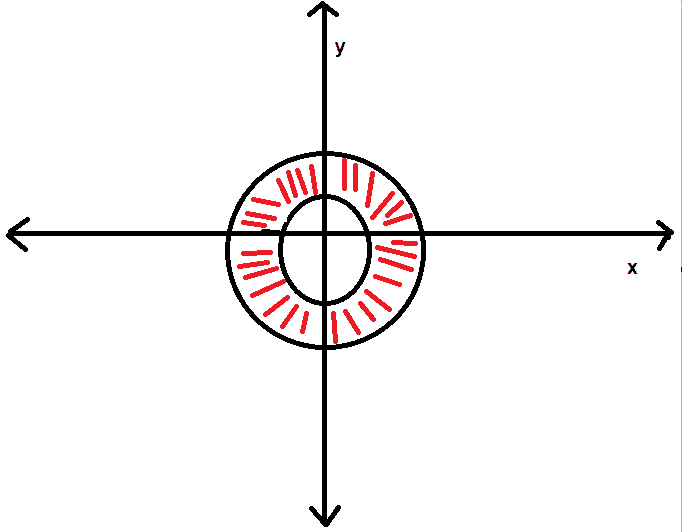I think the shaded region(drawn red lines) in the following graph is the line integral intended in this question.

Nope, the parametric curve defined by x = cos(t) and y = sin(t) where 0 ≤ t≤ 2π you must know!
Try to plot it for some values of t like 0, π/4, π/2, 3π/4, and so on...

Nope, the parametric curve defined by x = cos(t) and y = sin(t) where 0 ≤ t≤ 2π you must know!
Try to plot it for some values of t like 0, π/4, π/2, 3π/4, and so on...
Do you mean to say these curves?
y1(x)=cos(x)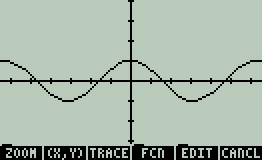y2(x)=sin(x)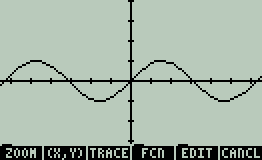Simultaneous plottings of both the graphs below: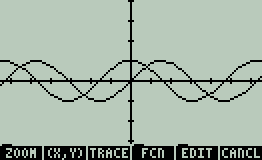No plot the points (x,y) for some values of t in the range from 0 to 2π, where x = cos t and y = sin t ...

I'll give you a hint, here are the points for t = π/4 and t = π/4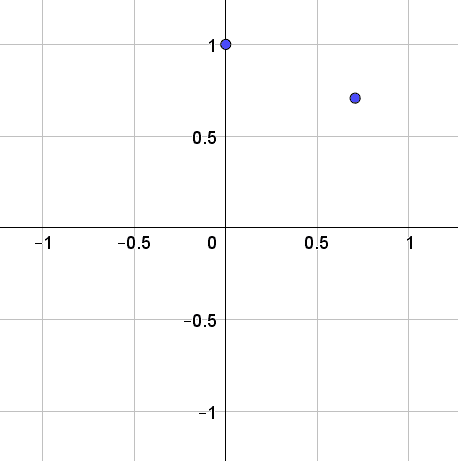How come you are doing line-integrals but don't know the basics of parametric curves?

No plot the points (x,y) for some values of t in the range from 0 to 2π, where x = cos t and y = sin t ...

I'll give you a hint, here are the points for t = π/4 and t = π/4

View attachment 303638

How come you are doing line-integrals but don't know the basics of parametric curves?
Do you mean the following graph?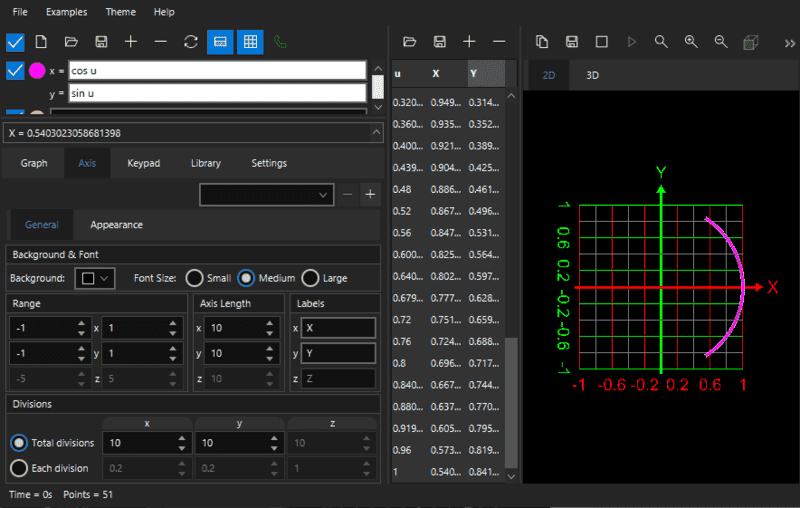What does the entire parametric curve C look like?

What does the entire parametric curve C look like?
Have you seen my post #15? In the graph given in #15 ,t is substituted by u.

Have you seen my post #15? In the graph given in #15 ,t is substituted by u.

the parameter values are not between 0 and 2π hence the entire curve C is not plotted

the parameter values are not between 0 and 2π hence the entire curve C is not plotted
In the following graph, is the entire curve C plotted?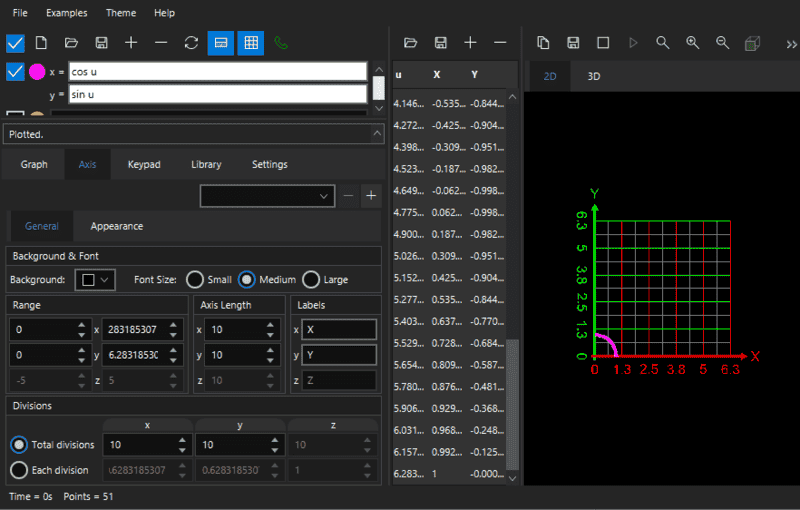Last edited:
u(t) ranges from 0 to 2π, so x = cos (u) will also range from -1 to 1 and y = sin(u) will also range from -1 to 1.

Now you got to be kidding me.

What if t = 3π/4? Then x<0 but y>0

I think you need to practice basics first.

Another way of analyzing that parametric curve is to see that x2 + y2 = 1 for all values of t. What kind of curve satisfies the equation x2 + y2 = 1?

Now you got to be kidding me.

What if t = 3π/4? Then x<0 but y>0

I think you need to practice basics first.

Another way of analyzing that parametric curve is to see that x2 + y2 = 1 for all values of t. What kind of curve satisfies the equation x2 + y2 = 1?
I am a novice in using the graphing calculator. I can plot it manually, but it is time consuming and cumbersome exercise. Thanks for helping me to answer this question.

I am a novice in using the graphing calculator. I can plot it manually, but it is time consuming and cumbersome exercise. Thanks for helping me to answer this question.

You really need to know how these curves work, rely on brain not on calculator.

here I did it for you with geogebra using the slider function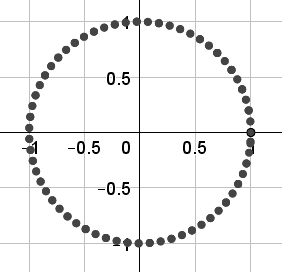now having tighter values of t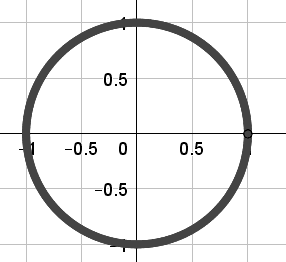looks to me its a circle with radius 1 and center at the origin. Wow that's so cool! But hey, the equation for such circle is x2 + y2 = 1, which is indeed satisfied for x = cos t and y = sin t

Bonus question, if t was ranging from 0 to π then the curve would become this: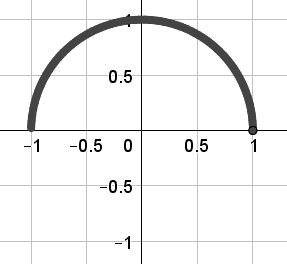this is becase we will never hit any negative y-values for this range of t, so the curve in this case is not closed, it is a semi-circle.

•WMDhamnekar
You really need to know how these curves work, rely on brain not on calculator.

here I did it for you with geogebra using the slider function

View attachment 303643
now having tighter values of t
View attachment 303645

looks to me its a circle with radius 1 and center at the origin. Wow that's so cool! But hey, the equation for such circle is x2 + y2 = 1, which is indeed satisfied for x = cos t and y = sin t

Bonus question, if t was ranging from 0 to π then the curve would become this:
View attachment 303646

this is becase we will never hit any negative y-values for this range of t, so the curve in this case is not closed, it is a semi-circle.
The graph you drawn is same which was posted in #11. But, I didn't write the x and y coordinates in that graph. It was the rough sketch I drawn(#11). Sorry, if you have any trouble while helping me to answer this question.

The graph you drawn is same which was posted in #11. But, I didn't write the x and y coordinates in that graph

It is not a circle that you draw, it looks like an "elliptic" version of an annulus https://en.wikipedia.org/wiki/Annulus_(mathematics)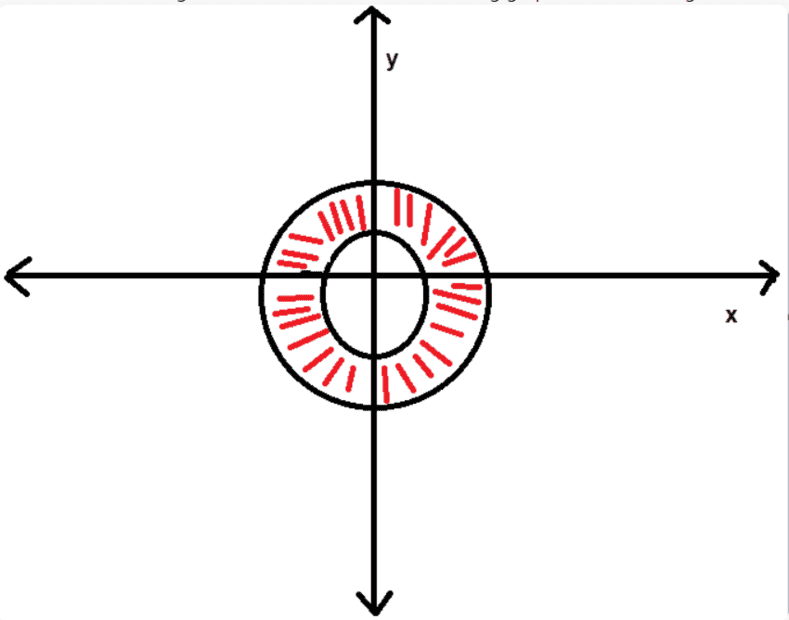It is not a circle that you draw, it looks like an "elliptic" version of an annulus https://en.wikipedia.org/wiki/Annulus_(mathematics)

View attachment 303648
Hi,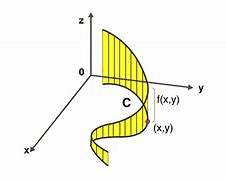If the above given graph is the line integral, how would you draw a line integral for the circle drawn by you?
That's what I did in #11 though it looks like the annulus as you said

Hi,
View attachment 303651
If the above given graph is the line integral, how would you draw a line integral for the circle drawn by you?
That's what I did in #11 though it looks like the annulus as you said

I only asked what the curve C looked like. You have to read things carefully.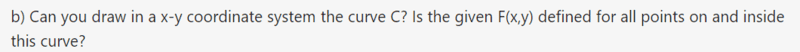and your function f(x,y) is a VECTOR function, it takes a coordinate as input and spits out a vector.•malawi_glenn
How to prove C f∙ dr =0 in the example given below: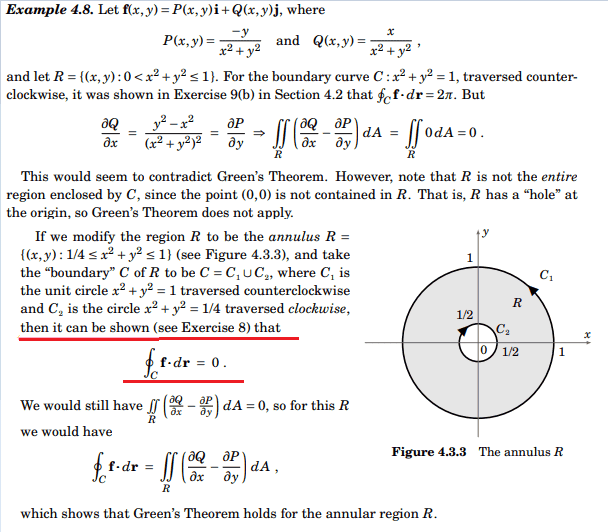Last edited:
•Delta2 and dextercioby
How to prove ∮C f∙ dr =0 in the example given below:

That result depends on what region R you are considering.

Here, first the origin is removed so that Greens theorem does not apply. R is all points (x,y) s.t. 0 < x2 + y2 ≤ 1. Thus the curve C: x = cos t, y = sin t, 0 ≤ t ≤ 2π does not enclose the entire region R. C covers more than R. This means that Greens theorem is not applicable.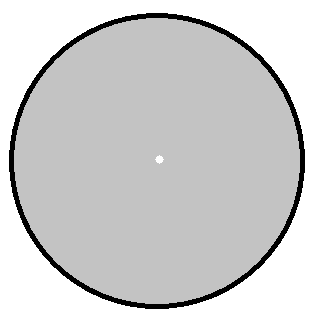Then they change the region so that there is an annulus instead, and now Greens theorem applies.

The problem as you originallly phrased it, did not mention any region whatsoever, only a curve. And when you do so, then it is implied that the region R is everything on and inside of C.

You have to pay attention to these little details, they are crucial.

Author has not proved in this example, how Cf∙dr = C1f∙dr1 +C2 f∙dr2 = 0.
I want to know how can we prove it?

( I think author left this topic for the readers to prove themselves as a homework exercise)

•Delta2
What is C1 and C2 and how are those related to C?
Is f a general vector function?

What is C1 and C2 and how are those related to C?
Is f a general vector function?
I think for both C1 and C2, x= cos(t), y=sin(t) where 0≤t≤2π and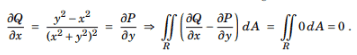and that's why
C1f⋅dr = C2f⋅dr =0.
Am I correct?

I think for both C1 and C2, x= cos(t), y=sin(t) where 0≤t≤2π and

Eh no, C1 and C2 must be related to C in some way and C must be a general closed curve. It should be written in your book. Btw are you sure they should be closed? indicates so, but are you really sure the question is formulated in that way? Otherwise, if they are closed, they should be related like this (but I do not know for sure since you are not providing enough info)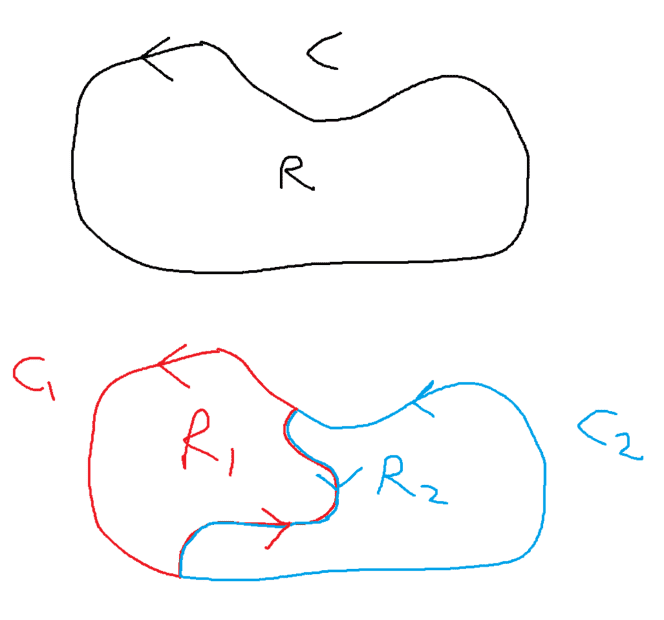Eh no, C1 and C2 must be related to C in some way and C must be a general closed curve. It should be written in your book. Btw are you sure they should be closed? indicates so, but are you really sure the question is formulated in that way? Otherwise, if they are closed, they should be related like this (but I do not know for sure since you are not providing enough info)
View attachment 303692
Whatever information you require is given in this example and related figure.For more general proof, you read below given study material.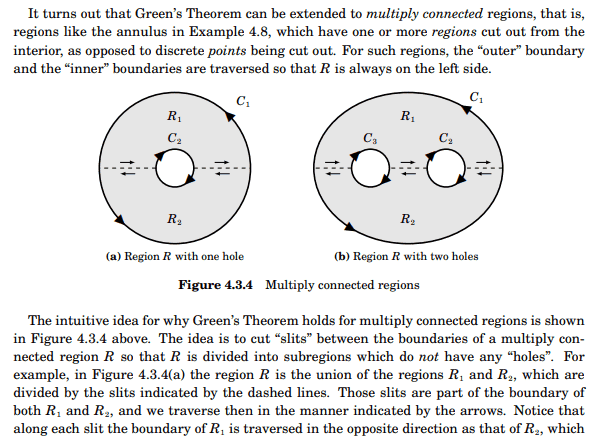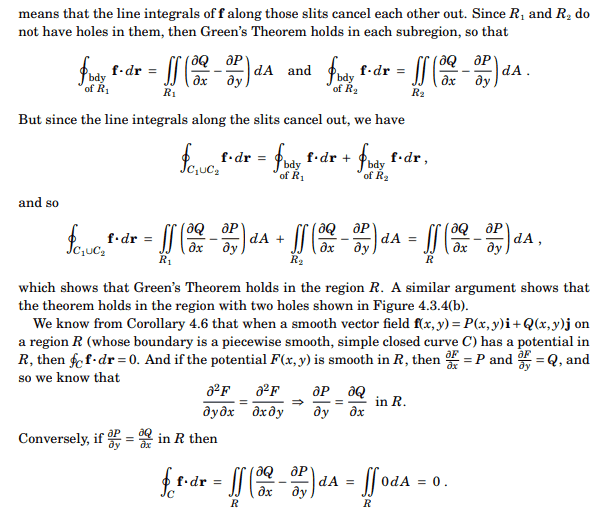Last edited:
So it is not a general theorem or such? Just for this example? Then you just calculate:
C1f⋅dr1 which is equal to 2π
and
C2f⋅dr2 which is equal to -